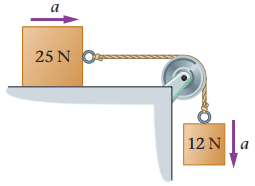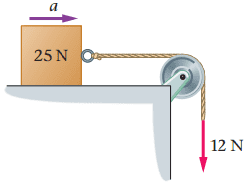# Find the magnitude of the acceleration of the two blocks.

## Homework Statement

a)Find the magnitude of the acceleration of the two blocks in (Figure 1) . Assume the top block slides without friction on the horizontal surface.

b)If the 12-N block in (Figure 1) is replaced with a 12-N force pulling downward, as in (Figure 2) , is the acceleration of the sliding block greater than, less than, or equal to the acceleration calculated in part A?
Less than
Greater than
Equal to
c)Calculate the acceleration of the block in (Figure 2) , assuming it slides without friction.## The Attempt at a Solution

I have attached a picture of my free body diagram. But I don't know where to start after that. I am thrown off because the number in the boxes are not masses.

#### Attachments

PhanthomJay
Homework Helper
Gold Member
The numbers in the blocks are the weights of the blocks , w= mg. It is confusing when the problem is shown that way . But you can solve for m, then continue.

Chestermiller
Mentor
In order for the 12 N block to accelerate downward, does the tension in the string have to be greater than 12 N, less than 12 N, or equal to 12 N?

The numbers in the blocks are the weights of the blocks , w= mg. It is confusing when the problem is shown that way . But you can solve for m, then continue.
okay so m=w/g?

PhanthomJay
Homework Helper
Gold Member
Yes

•Angela_vaal
In order for the 12 N block to accelerate downward, does the tension in the string have to be greater than 12 N, less than 12 N, or equal to 12 N?
less than 12 N? Because If it is greater wouldn't it make it go upward?

Figure 2.Chestermiller
Mentor
less than 12 N? Because If it is greater wouldn't it make it go upward?

okay so I figured out the magnitude of the acceleration of the two blocks. I am a bit confused how to do part b. I have attached a picture of my FBD and equations. I would like to know if the second equation is right. If it is or is not equal to "0" since there is no mass. And if there is no mass it would also means there is no acceleration either.

#### Attachments

Never mind the work I did for equation 2 I did above as noted in the photo. my m2 is instead T=-12N. I plugged that into the first equation and got -4.7m/s2. for my first value I got 3.2 m/s2. would part b be less than ?

Chestermiller
Mentor
To get the answer to part b, all you need to do is compare the acceleration for part c with the acceleration for part a.

•Angela_vaal